# DAV Class 5 Maths Chapter 4 Worksheet 7 Solutions

The DAV Class 5 Maths Book Solutions Pdf and DAV Class 5 Maths Chapter 4 Worksheet 7 Solutions of Fractional Numbers offer comprehensive answers to textbook questions.

## DAV Class 5 Maths Ch 4 Worksheet 7 Solutions

Question 1.
Multiply the following fractional numbers.
(a) $$\frac{2}{3} \times \frac{4}{5}$$
Solution: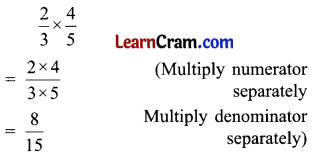(b) $$\frac{4}{7} \times \frac{1}{3}$$
Solution:(c) $$\frac{3}{8} \times \frac{5}{11}$$
Solution:(d) $$\frac{2}{5} \times \frac{15}{16}$$
Solution: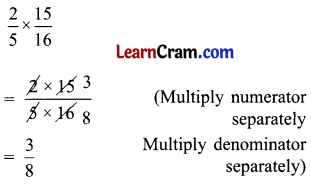(e) $$3 \frac{1}{4} \times \frac{8}{9}$$
Solution: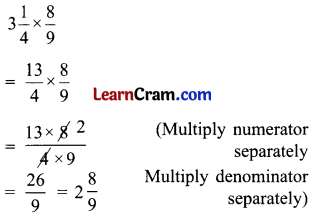(f) $$7 \frac{1}{2} \times 8 \frac{1}{3}$$
Solution:(g) $$2 \frac{2}{5} \times \frac{2}{15}$$
Solution:(h) $$\frac{6}{7} \times 3 \frac{1}{2}$$
Solution: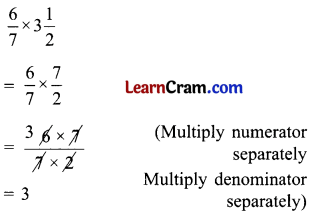(i) $$\frac{1}{10} \times \frac{2}{3} \times \frac{5}{8}$$
Solution:(j) $$1 \frac{2}{5} \times \frac{4}{21}$$
Solution: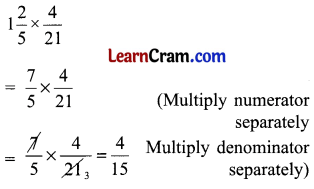(k) $$5 \frac{5}{6} \times 2 \frac{1}{7}$$
Solution:(l) $$\frac{4}{5} \times \frac{7}{8} \times \frac{24}{35}$$
Solution: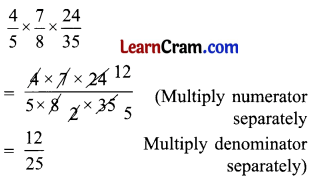DAV Class 5 Maths Chapter 4 Worksheet 7 Notes

Example 1.
Find $$\frac{3}{4}$$ of $$\frac{1}{2}$$.
Solution:Consider a rectangle. Make it half. Divide each part into four equal parts.
We want to find out $$\frac{3}{4}$$ of $$\frac{1}{2}$$. So we shade three parts of four parts of the half.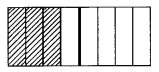The shaded portion is $$\frac{3}{8}$$ of the whole.
Hence, $$\frac{3}{4}$$ of $$\frac{1}{2}$$ is $$\frac{3}{8}$$.Example 2.
Let us multiply $$\frac{1}{5}$$ of $$\frac{2}{7}$$.
Solution:
$$\frac{1}{5} \times \frac{2}{7}=\frac{1 \times 2}{5 \times 7}$$ = $$\frac{2}{35}$$
(Multiply the numerator separately 35 Multiply the denominator separately)

Example 3.
Multiply $$2 \frac{2}{5}$$ and $$8 \frac{1}{2}$$
Solution: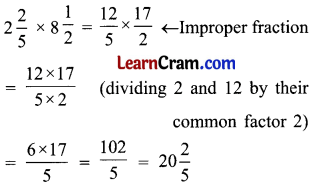Example 4.
Multiply $$\frac{1}{5}$$, $$\frac{5}{7}$$ and $$\frac{2}{3}$$.
Solution: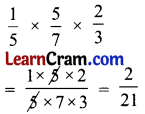Remember: In order to multiply two fractional numbers, we multiply the numerators and the denominators separately.